## Hartley Transform

An Integral Transform which shares some features with the Fourier Transform, but which (in the discrete case), multiplies the Kernel by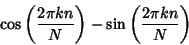(1)(2)

The Hartley transform produces Real output for a Real input, and is its own inverse. It therefore can have computational advantages over the Discrete Fourier Transform, although analytic expressions are usually more complicated for the Hartley transform.

The discrete version of the Hartley transform can be written explicitly as(3)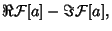(4)

where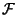denotes the Fourier Transform. The Hartley transform obeys the Convolution property(5)

where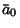(6)(7)(8)

(Arndt). Like the Fast Fourier Transform, there is a fast'' version of the Hartley transform. A decimation in time algorithm makes use of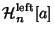(9)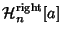(10)

wheredenotes the sequence with elements(11)

A decimation in frequency algorithm makes use of(12)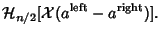(13)(14)

can be written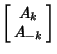(15)(16)

so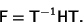(17)

References

Arndt, J. The Hartley Transform (HT).'' Ch. 2 in Remarks on FFT Algorithms.'' http://www.jjj.de/fxt/.

Bracewell, R. N. The Fourier Transform and Its Applications. New York: McGraw-Hill, 1965.

Bracewell, R. N. The Hartley Transform. New York: Oxford University Press, 1986.